# WBJEE 2016 Chemistry Paper with Solutions

WBJEE 2016 Chemistry paper with simple and understandable solutions are provided on this page. The PDF form of the solutions is also available here for download. Practising previous years’ questions will help the candidates get familiar with the pattern of the question paper and gain confidence in solving the problems. Students are advised to download the WBJEE 2016 Chemistry paper and practise offline during their study hours.

### WBJEE 2016 - Chemistry

Question 1: Consider the following compounds: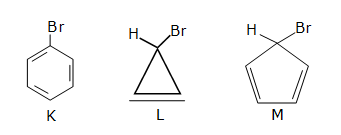1. a. Only K forms a precipitate on treatment with alcoholic AgNO3 solution.
2. b. Only L forms a precipitate on treatment with alcoholic AgNO3 solution.
3. c. Only M forms a precipitate on treatment with alcoholic AgNO3 solution.
4. d. K, L, and M form precipitates with alcoholic AgNO3 solution.

Solution:

Those compounds will easily form a precipitate with AgNO3 in which the conjugate formed is stable.

In option L, we can see that the compound formed is aromatic in nature i.e. contains

(4n + 2)π conjugation so is stable.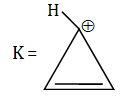This compound is stable since it is aromatic.

Question 2: Consider the following nuclear reactions $${ }_{92}^{238} M \rightarrow{ }x^Y N+2 \alpha ; _{x}^{y}N \rightarrow{ }_{{B}}^{{A}} {L}+2 \beta^{+}$$: The number of neutrons in the element L is

1. a. 142
2. b. 144
3. c. 140
4. d. 146

Solution:

Nuclear reaction is:-

$${ }_{92}^{238} M \rightarrow{ }x^Y N+2 \alpha(2\times{ }_{2}^{4} He ) or{ }_{88}^{230} N$$

$${ }_{88}^{230} N \rightarrow{ }_B^A L+2 { }_{1}^{0} e \, or\, { }_{96}^{230} L$$

Therefore, Number of neutrons in L = 230 – 36

= 144

Question 3: Of the following atoms, which one has the highest n/p ratio?

1. a. Ne16
2. b. O16
3. c. F16
4. d. N16

Solution:

Highest n/p ratio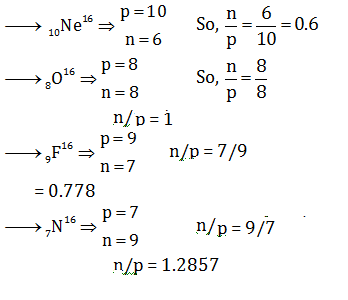So, the highest n/p ratio is of N16

Question 4: The spin-only magnetic moment of [CrF6]4– (atomic number of Cr is 24) is

1. a. 0
2. b. 1.73 BM
3. c. 2.83 BM
4. d. 4.9 BM

Solution: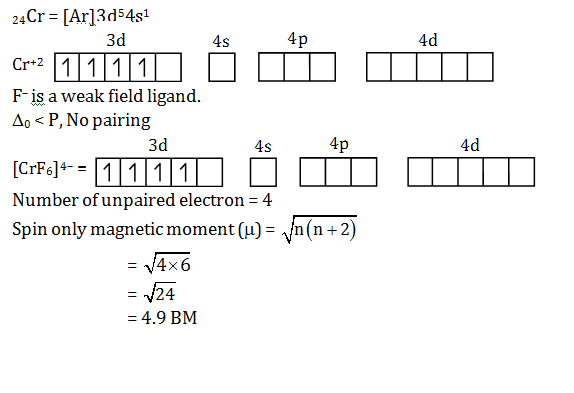Question 5: Among the following groupings, which one represents the set of iso-electronic species?

1. a. NO+, C22–, O2, CO
2. b. N2, C22–, CO, NO
3. c. CO, NO+, CN, C22–
4. d. NO, CN, N2, O2

Solution:

Two or more molecular entities are as iso-electronic if they have the same number of valance electrons and same structure i.e. number and connectivity of atoms.

Here we can see that CO, NO+, CN, C22_

All have 14 electrons each.

Carbon has 6 electrons, oxygen has 8 electrons, nitrogen has 7 electrons.

Question 6: In the complex ion [Cu(CN)4]3– the hybridization state, oxidation state and number of unpaired electrons of copper are respectively

1. a. dsp2, +1,1
2. b. sp3, +1, zero
3. c. sp3, +2,1
4. d. dsp3, +2, zero

Solution: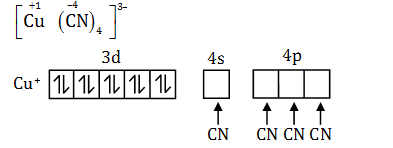Hybridization = sp3

Oxidation state of Cu = +1

Number of unpaired electrons = 0.

Question 7: The maximum number of 2p electrons with electronic spin =-1/2 are

1. a. 6
2. b. 0
3. c. 2
4. d. 3

Solution:

The maximum electrons which can be accommodated in p-orbital is 6 electrons.

Three electrons have +1/2 spin and other three have –1/2 spin.

So, a maximum electron in 2p with a spin –1/2 is 3.

Question 8: For N3– > O2– > F and Na+, the order in which their ionic radii varies is

1. a. N3– > O2– > F > Na+
2. b. N2– > Na+ > O 2– > F
3. c. Na+ > O 2– > N 3– > F
4. d. O2– > F > Na + > N3–

Solution:

N3–, O2–, F, Na+ all have the same number of valance electrons. So, these species are isoelectronic and we know, as atomic number increases. Nuclear attraction increase, hence the order of ionic radii will be

N 3– > O2– > F > Na+

Question 9: Assign the Bravais lattice type of the following unit cell structure.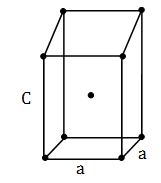1. a. Cubic I
2. b. Orthorhombic I
3. c. Tetragonal I
4. d. Monoclinic

Solution:

By seeing the given structure. We can conclude that a = b ≠ c and all angles are 90° i.e.

α = β = γ = 90°

And it is body centred lattice i.e. the Bravais notation is I.

Therefore, Bravais lattice is Tetragonal-I.

Question 10: The equilibrium constant for the reaction N2+3H2 $$\rightleftharpoons$$ 2NH3 is ‘K’. Then, the equilibrium constant for the reaction 2N2+6H2 $$\rightleftharpoons$$ 4NH3 will be

1. a. K
2. b. K2
3. c. $$\sqrt{K}$$
4. d. 2K

Solution:

Given: N2+3H2 $$\rightleftharpoons$$ 2NH3

equilibrium constant = k

Multiplying equation by 2, we get

2N2+6H2 $$\rightleftharpoons$$ 4NH3

If equation is multiplied by 2 then k will be squared K2

Or

N2 + 3H2$$\rightleftharpoons$$ 2NH3, K

$$K=\frac{\left [ NH_{3} \right ]^{2}}{\left [ N_{2} \right ]\left [ H_{2} \right ]^{3}}$$

Now

2N2+6H2 $$\rightleftharpoons$$ 4NH3

$$K'=\frac{\left [ NH_{3} \right ]^{4}}{\left [ N_{2} \right ]^{2}\left [ H_{2} \right ]^{6}}$$=K2

Question 11: Which of the following is the correct option for free expansion of an ideal gas under adiabatic condition?

1. a. q = 0, ΔT ≠0, W = 0
2. b. q = 0, ΔT ≠ 0, W ≠0
3. c. q = 0, ΔT =0, W = 0
4. d. q = 0, ΔT = 0, W ≠ 0

Solution:

For the free expansion of real gas, the opposing force is zero, and when opposing force is zero then work done = 0

We, know the equation

ΔU = q + w

If w= 0 then ΔU = q

But the process is adiabatic hence q = 0

Therefore, q = 0, T = 0, w = 0

Question 12: 75% of a first order reaction was completed in 32 min. When would 50% of the reaction complete?

1. a. 24 min
2. b. 16 min
3. c. 8 min
4. d. 64 min

Solution: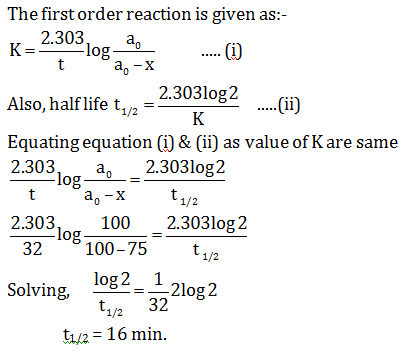Question 13: Pressure (P) vs. density (D) curve for an ideal gas at two different temperatures T1 and T2 is shown below.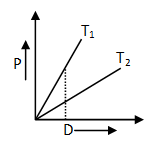1. a. T1 > T2
2. b.T1 < T2
3. c. T1 = T2
4. d. Cannot be said

Solution:

As we know,

Ideal gas equation is PV = nRT

$$P=\frac{wRT}{MV}$$ or we can write

So,

PM = dRT

P ∝ d.T

So, T1 > T2

Question 14: Which of the following compounds is least effective in precipitating Fe(OH)3 Solution:?

1. a. K4[Fe(CN)6]
2. b. K2CrO4
3. c. KBr
4. d. K2SO4

Solution:

Fe(OH)3 is positively charged Solution: charge of Br anion is least and so KBr is least effective in coagulating Fe(OH)3 Solution:

Also, for coagulating positive charged Solution: negatively charged Solution is required. More the negative charge, more easily the coagulation will take place.

Question 15: Which statement is incorrect?

1. a. Borazine has a 3D-layer structure like that of graphite
2. b. Boric acid has a hydrogen-bonded layer structure in the solid-state
3. c. Borazine molecule is (BN)3
4. d. [Al6O18]18– contains a non-planar Al6O6-ring

Solution:

Three options are incorrect.

Borazine is B3N3H6 and its structure is not like that of graphite.

[Al6O18] –18 contain tetrahedral units.

Question 16: Which one of the following does not produce O2 as the only gaseous product on heating?

2. b. Potassium Chlorate
3. c. Mercuric Oxide
4. d. Potassium Nitrate

Solution:

We can find out the answer by writing heating equations of compounds.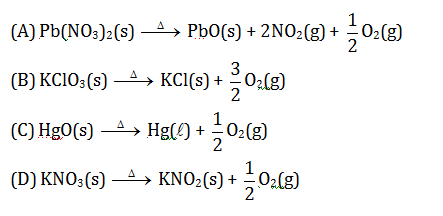Therefore, we can observe that when lead nitrate is heated it will give NO2 gas along with O2 gas.

Question 17: Which of the following is true in respect to adsorption?

1. a. ΔG < 0; Δ> 0 ; ΔH < 0
2. b. ΔG < 0; ΔS < 0; ΔH < 0
3. c. ΔG > 0; ΔS> 0 ; ΔH < 0
4. d. ΔG < 0; ΔS <0; ΔH > 0

Solution:

∆G < 0, ∆H < and ∆S < 0

$$\rightarrow$$Adsorption of gases on solid surface is generally exothermic because entropy decreases.

$$\rightarrow$$Adhering of gas molecules to the surface lowers randomness.

Question 18: Which property that polyacetylene exhibit is unusual for an organic polymer?

1. a. Electrical conductivity
2. b. Flexibility
3. c. High boiling point
4. d. Solubility

Solution:

Due to the conjugation, it can conduct electricity.

The structure of polyacetylene is shown:Question 19: Which statement is incorrect about complexes formed by the lanthanoids?

1. a. Hard donor ligands are favoured
2. b. High coordination numbers (more than six) are often observed
3. c. The 4f atomic orbitals do not play a significant part in metal-ligand bonding
4. d. Aqua ions are typically 6-coordinate

Solution:

The statement aqua ions typically 6-coordinate is incorrect, for example, the coordination number for [Ln(H2O)n]3+ in aqueous solution is thought to be 9 for the early lanthanoids and for the later, smaller numbers of the series.

Question 20: In the alumino-thermite process, aluminium acts as

1. a. a reducing agent
2. b. an oxidizing agent
4. d. a flux

Solution:

Some metal oxides cannot be reduced satisfactorily by carbon. For them, aluminium which is a more reactive metal is used. The process is called thermic process or alumino-thermic process since this process is only for those metals that can’t be reduced by carbon. Therefore, aluminium is used in place of carbon atoms and it acts as a reducing agent.

Aluminium reduces oxides of metals like Fe/Cr/Mn at elevated temperature.

2Al + Fe2O3 $$\rightarrow$$ 2Fe + Al2O3

Question 21: Consider the following reaction: 6NaOH + 3Cl2 $$\rightarrow$$5NaCl + A + 3H2O what is the oxidation number of chlorine in A?

1. a. +5
2. b. –1
3. c. +3
4. d. +1

Solution:

Sodium hydroxide reacts with chlorine gives sodium chloride, sodium chlorate and water molecules as the products. The chemical reaction can be written as:

6NaOH + 3Cl2 $$\rightarrow$$ 5NaCl + 3H2O + NaClO3

(A)

So, the compound (A) is NaClO3

Let the oxidation state of chlorine is x.

The oxidation state of Cl in NaClO3

(+1) + (x) + 3(–2) = 0

x = 6 –1

x = +5

Oxidation number of chlorine is +5.

Question 22: A sudden large difference between the values of second and third ionization energies of elements would be associated with which of the following electronic configurations?

1. a. 1s2 2s2 2p6 2s1
2. b. 1s2 2s2 2p6 3s2
3. c. 1s2 2s2 2p6 3s2 3p1
4. d. 1s2 2s2 2p6 3s2 3p2

Solution:

After removal of two electrons from 1s2, 2s2, 2p6, 3s2 we get stable noble gas (Ne) configuration. On removing one more electron in this completely filled octet, a high amount of energy required. So there is a large difference between 2nd ionization energy and 3rd ionization energy for this configuration.

Question 23: Na2O2 is produced in the reaction between H2O2 and NaOH. Here, the role of H2O2 is

1. a. as an oxidising agent
2. b. as an acid
3. c. as a base
4. d. as a reducing agent

Solution:

It is an acid-base reaction in which hydrogen peroxide act as acid to react with sodium hydroxide (base) to produce sodium peroxide and water.

Question 24: Amongst the following compounds, the one which would not form a white precipitate with ammoniacal silver nitrate solution is

1. a. HC ≡CH
2. b.H3C – C ≡ C – CH3
3. c. H3C – C ≡ CH
4. d.CH3CH2CH2C ≡ CH

Solution:

Only terminal alkynes having acidic hydrogen to react with ammoniacal silver nitrate solution known as Tollen’s reagent.

In the given options, CH3≡CCH3 does not have any acidic hydrogen to react with an ammoniacal silver nitrate solution

Question 25: m-dinitrobenzene can be converted to m-nitroaniline by reduction with:

1. a. Raney Nickel
2. b. LiAlH4
3. c. (NH4)2S
4. d. Na/C2H5OH

Solution:

Selective reduction of m-nitro benzene to form m-nitroaniline is done by ammonium sulphide. In this reaction out of two nitro groups, only one –NO2 group is reduced.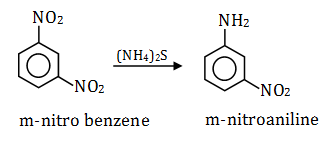Question 26: The correct IUPAC name of H3C – C(CH3)2 – CH = CH2 is :

1. a. 3, 3, 3– trimethylprop–1–ene
2. b. 1, 1,1 trimethyl- α–propene
3. c. 3, 3–dimethylbut–1–ene
4. d. 2, 2–dimethylbut–3–ene

Solution:

The correct IUPAC name of H3C–C(CH3)2–CH=CH2 is 3,3-dimethyl but-1-ene.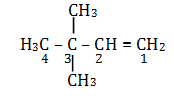Question 27: Which combination of reagents used in the indicated order will give m-nitropropyl benzene from benzene?

1. a.1) conc. HNO3/conc. H2SO4

2) CH3CH2CH2/AlCl3

2. b. 1)CH3CH2CH2Cl/AlCl3

2) conc. HNO3/conc. H2SO4

3. c. 1) CH3CH2COCl/AlCl3

2) conc. HNO3/conc. H2SO4

3) H2NNH2/NaOH

4. d. 1) conc. HNO3/conc. H2SO4

2) CH3CH2COCl/AlCl3

3) H2NNH2/NaOH

Solution:

Correct order to form m-nitropropyl benzene from benzene: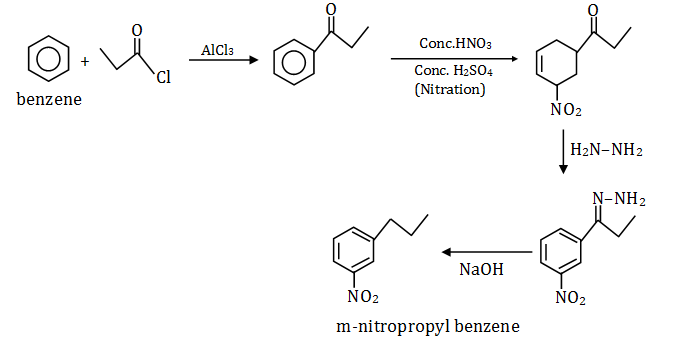Question 28: Which of the statement (A) - (D) about the reaction profile below is false?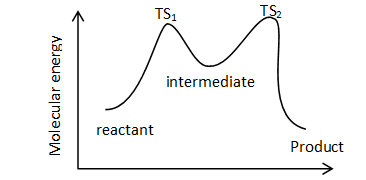1. a. The product is more stable than the reactant
2. b. The second step is rate determining
3. c. The reaction is exothermic
4. d. The equilibrium constant is greater than 1 if the molar entropy change is negligible

Solution:

The potential energy diagram shows the lower activation energy of a hypothetical reaction in the second step.

The rate of reaction depends on the activation energy; lower activation energy means that a larger number of molecules will have sufficient energy to undergo an effective collision.

Hence, the second step is not a rate-determining step, it is a fast step due to low activation energy.

Question 29: Which of the following is the major product when one mole of propanone and two moles of benzaldehyde react in presence of a catalytic amount of NaOH?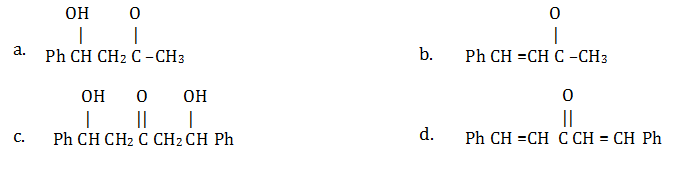Solution:

This reaction is an example of aldol condensation reaction and is given by aldehydes or ketones having α-H atom to give β-hydroxy aldehyde.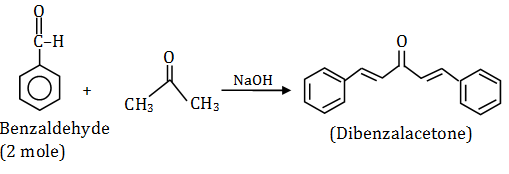Question 30: For the following anion,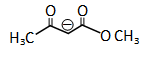the resonance structure that contributes most is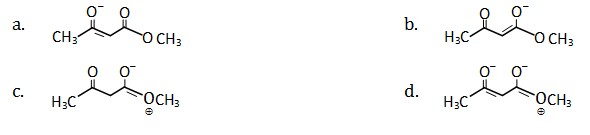Solution: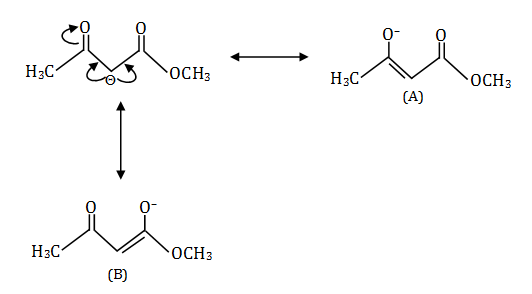Resonance hybrid (A) is more stable than (B)

Option c and d is least stable because of the positive charge on the electronegative oxygen atom.

Question 31: The major product obtained upon treatment of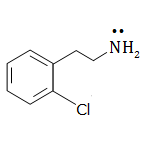with NaNH2 and liquid NH3 is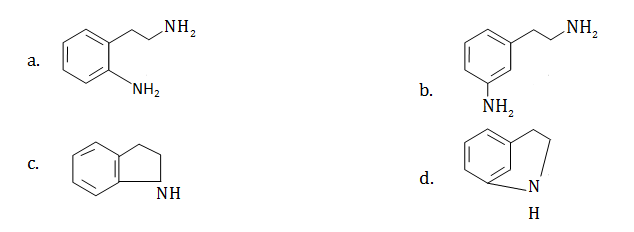Solution: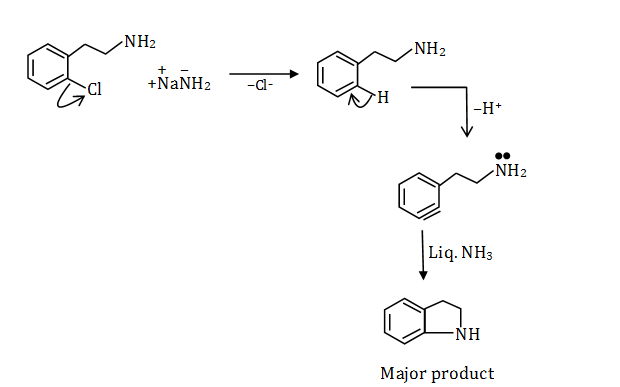Question 32: Which structure for XeO3 and XeF4 is consistent with the VSEPR model?

1. a. XeO3, trigonal pyramidal; XeF4, square planar
2. b. XeO3, trigonal planar; XeF4 square planar
3. c. XeO3, trigonal pyramidal; XeF4, tetrahedral
4. d. XeO3, trigonal planar; XeF4, tetrahedral

Solution:

Xe has electronic configuration [Kr]5s2 5p6.

In the case of XeF4, the structure will be square planar. There are four pairs of bonding electrons and two lone pair in the molecule. Two lone pairs will be opposite each other to maintain symmetry.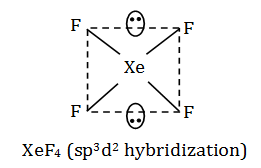XeO3 has trigonal pyramidal geometry with asymmetric charge distribution on central atom. There are three pairs of bonding electrons and one lone pair in the molecule.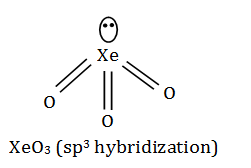Question 33: If CO2 gas is passed through 500 ml of 0.5 (M) Ca(OH)2, the amount of CaCO3 produced is of the value

1. a. 10 g
2. b. 20 g
3. c. 50 g
4. d. 25 g

Solution:

CO2 + Ca(OH)2 $$\rightarrow$$CaCO3 + H2O

Given, volume of Ca(OH)2 = 500 mL

Concentration of Ca(OH)2 = 0.5 M

Moles of Ca(OH)2 = Volume(v) × concentration

=[(500x0.5)/1000] = 0.25 mole

1 mole Ca(OH)2 gives 1 mole CaCO3

0.25 mole Ca(OH)2 gives =(1/1) × 0.25 mole CaCO3 = 0.25 mole CaCO3

Mass of CaCO3 = mole × molar mass {Therefore, Molar mass of CaCO3 is 100 g/mole}

= 0.25 mole × 100 g/mole = 25 g

Question 34: The emf of a Daniel cell at 298 K is E1. The cell is Zn|ZnSO4 (0.01M) || CuSO4 (1M) | Cu When the concentration of ZnSO4 is changed to 1M and that of CuSO4 to 0.01M, the emf changes to E2. The relationship between E1 and E2 will be

1. a. E1 – E2 = 0
2. b. E1<E2
3. c. E1 > E2
4. d. E1 = 102E2

Solution:

Zn|ZnSO4(0.01m)||CuSO4(1m)|Cu

According to the Nernst equation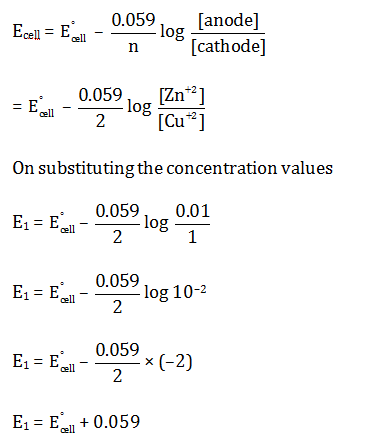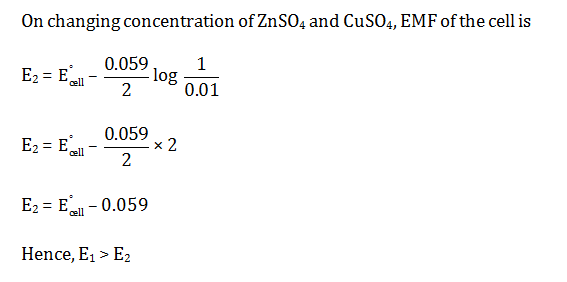Question 35: Which reaction is not appropriate for the synthesis of the following?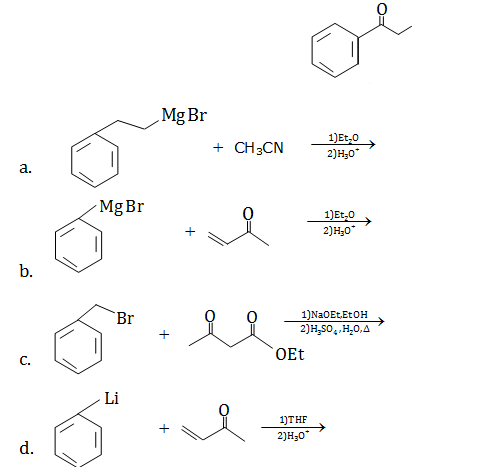Solution: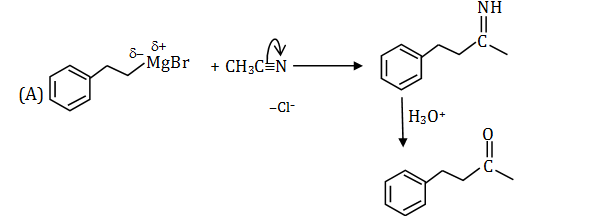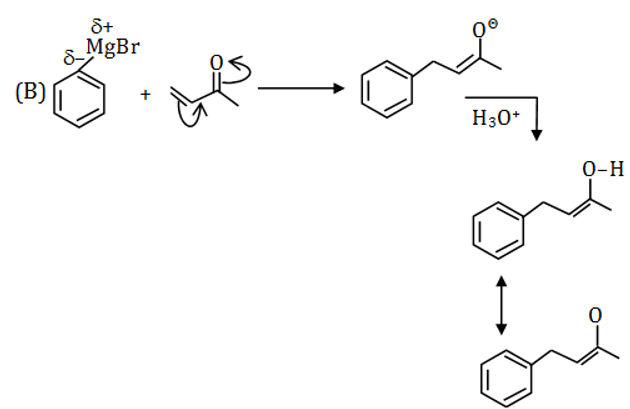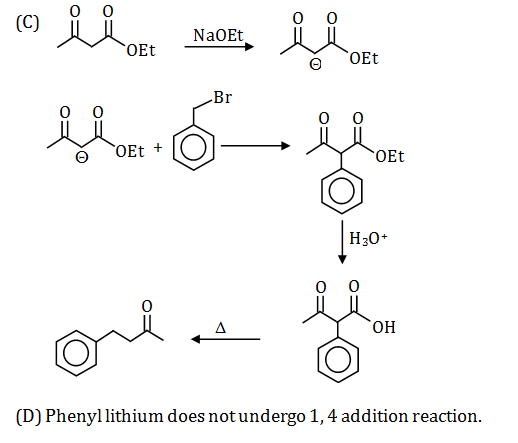Question 36: Which of the following statements are correct with reference to the isoelectric point of alanine?

1. a. At the isoelectric point, alanine bears no net charge
2. b. At the isoelectric point, the concentration of zwitterion is maximum
3. c. It is not the average of pKa1 and pKa2 value
4. d. Alanine will have a net positive charge at pH below the isoelectric point

Solution:

At the isoelectric point, the amino acid exists as zwitterion to the maximum ion concentration.

A zwitterion is a dipolar ion, i.e.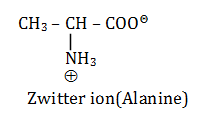For amino acids that have no ionisable side chain, the pI value is the average of its two pKa’s. If the amino acid has an ionisable side chain, the pI value is the average of the pKa’s of similarly ionisable groups.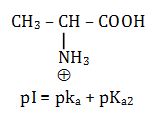Alanine has a net positive charge at pH below the isoelectric point.

Question 37: Consider the proposed mechanism for the destruction of ozone in the stratosphere?

O3 + Cl $$\rightarrow$$ ClO + O2

ClO + O3 $$\rightarrow$$ Cl + 2O2

Which of the statements about the mechanism is/are correct?

1. a. Cl is a catalyst
2. b. O2 is an intermediate
3. c. Equal amounts of Cl and ClO are present at any time
4. d. The number of moles of O2 produced equals the number of moles of O3 consumed

Solution:

(A) Electronic configuration of 24Cr: [Ar] 3d5 4s1, Due to extra stability.

(B)Magnetic quantum number may have a negative value.

When l = 2, m = –2, –1, 0, +1, +2

(C)Silver atoms have atomic number 47.

Question 38: Which of the following statements(s) is (are) correct?

1. a. The electronic configuration of Cr (at. no: 24) is [Ar] 3d5 4s1.
2. b. The magnetic quantum number may have a negative value.
3. c. In Ag (at. no: 47), 23 electrons have spins of one type and 24 electrons have spins of the opposite type.
4. d. The oxidation state of nitrogen in HN3 is – 3

Solution:

Electronic configuration 47Ag: 1s22s22p63s23p63d104s24p6 4d105s1

So, 23 electrons have a spin of one type and 24 of the opposite type.

In HN3, the oxidation state of N is -1/3.

Question 39: Equal quantities of electricity are passed through 3 voltmeters containing FeSO4, Fe2(SO4)3 and Fe(NO3)3. Consider the following statement:

(1) The amounts of iron deposited in FeSO4 and Fe2(SO4)3 are equal

(2) The amount of iron deposited in Fe(NO3)3 is 2/3rd of the amount deposited in FeSO4

(3) The amount of iron deposited in Fe2(SO4)3 and Fe(NO3)3 are equal

1. a. (1) is correct
2. b. (2) is correct
3. c. (3) is correct
4. d. Both (1) and (2) are correct

Solution:

1.FeSO4 $$\rightarrow$$Fe2+ + SO42–

Fe2+ + 2e $$\rightarrow$$ Fe(s)

Therefore, 2F charge is needed for 1 mole deposition of Fe.

2. Fe2(SO4)3 $$\rightarrow$$ 2Fe3+ + 3SO42–

Fe3+ + 3e $$\rightarrow$$ Fe(s)

3F $$\rightarrow$$1 mole

2F charge is needed for (2/3) mole deposition of Fe.

3. Fe(NO3)3$$\rightarrow$$ Fe3+ + 3NO3-

Fe3+ + 3e $$\rightarrow$$ Fe(s)

3F $$\rightarrow$$ 1 mole

2F charge is needed for 2/3 mole deposition of Fe.

Hence, the correct option is B & D.

Question 40: Which of the following statements are correct for the following isomeric compounds I and II?

1. a. I and II are enantiomers
2. b. I and II are both optically active
3. c. I is D-alanine while II is L-alanine
4. d. I and II are diastereomers

Solution: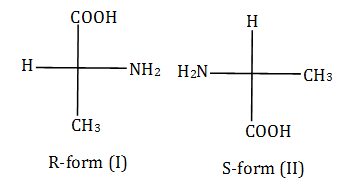Enantiomers: non-super imposable non-mirror images are enantiomers. So, compound I and II are enantiomers.

Compound I and II are optically active due to absence of plane of symmetry.

Compound I is D-alanine while II is L-alanine

### WBJEE 2016 Chemistry Question Paper with Solutions